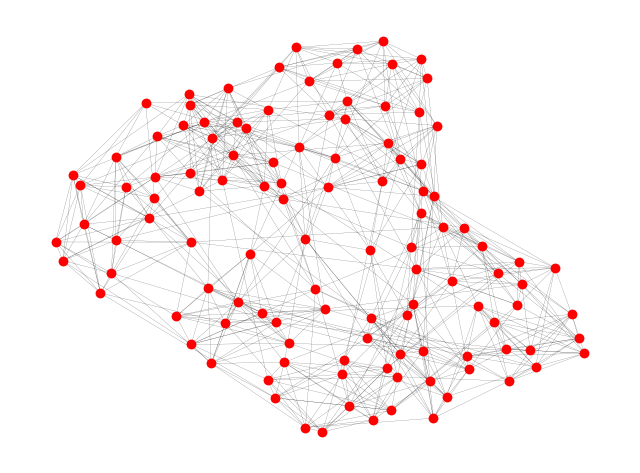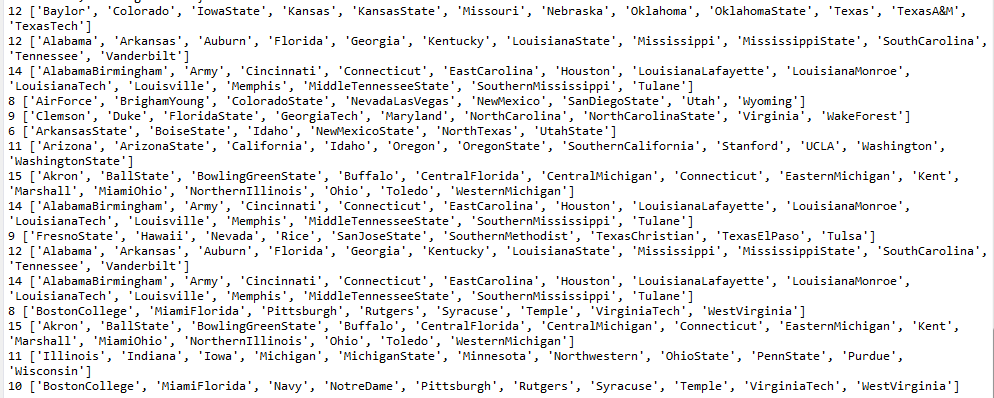• 社区发现算法python代码
2021-08-25 09:26:43

最近一直在学习python语言，之前一直使用matlab。看了很多博客都写了该算法的代码，我觉得还不具体，不详细。对于初学者来说，看懂不容易。写了一个NMF算法的python程序供大家参考。参照文献《Algorithms for non-negative matrix factorization》

具体代码如下：

import numpy as np
import torch
import random
import matplotlib.pyplot as plt

def nmf(X,r,maxiter,minError):
# X=U*V'
row,col = X.shape
U = np.around(np.array(np.random.rand(row, r)),5)
V = np.around(np.array(np.random.rand(col, r)),5)
obj = []
for iter in range(maxiter):
print('-----------------------------')
print('开始第',iter,'次迭代')
# update U
XV = np.dot(X,V)
UVV = np.dot(U,np.dot(V.T,V))
U = (U*(XV/np.maximum(UVV,1e-10)))
# update V
XU = np.dot(X.T,U)
VUU = np.dot(V,np.dot(V.T,V))
V = (V*(XU/np.maximum(VUU,1e-10)))
d = np.diag(1/np.maximum(np.sqrt(np.sum(V*V,0)),1e-10))
V = np.dot(V,d)

temp = X - np.dot(U,np.transpose(V))
error = np.sum(temp*temp)
print('error:',error)
print('第',iter,'次迭代结束')
obj.append(error)
if error<minError:
break
return U, V, obj

if __name__ =="__main__":
X = np.random.randn(20, 50)
X = np.array(np.abs(X))
#print('X:',X)
U,V,obj = nmf(X,2,100,0.01)
x = range(len(obj))
plt.plot(x, obj)
plt.show()python
更多相关内容
• 基于LFM的重叠社区发现算法python代码实现 import random import networkx as nx import matplotlib.pyplot as plt import zipfile #import urllib.request as urllib class Community(): ''' use set operation to...

## 基于LFM的重叠社区发现算法python代码实现

import random
import networkx as nx
import matplotlib.pyplot as plt
import zipfile
#import urllib.request as urllib
class Community():
''' use set operation to optimize calculation '''

def __init__(self,G,alpha=1.0):
self._G = G
self._alpha = alpha
self._nodes = set()
self._k_in = 0
self._k_out = 0
neighbors = set(self._G.neighbors(node))
#print("添加令居节点",neighbors , self._nodes,neighbors & self._nodes)
node_k_in = len(neighbors & self._nodes)#neighbor和self._nodes公有节点的数目存入node_k_in
#print("node_k_in",node_k_in)
node_k_out = len(neighbors) - node_k_in
#print("node_k_out",node_k_out)
self._k_in += 2*node_k_in
self._k_out = self._k_out+node_k_out-node_k_in

def remove_node(self,node):
neighbors = set(self._G.neighbors(node))
community_nodes = self._nodes
#print("community_nodes",community_nodes)
node_k_in = len(neighbors & community_nodes)
node_k_out = len(neighbors) - node_k_in
self._nodes.remove(node)
self._k_in -= 2*node_k_in
self._k_out = self._k_out - node_k_out+node_k_in

neighbors = set(self._G.neighbors(node))
old_k_in = self._k_in
old_k_out = self._k_out
vertex_k_in = len(neighbors & self._nodes)#vertex顶点
vertex_k_out = len(neighbors) - vertex_k_in
new_k_in = old_k_in + 2*vertex_k_in
new_k_out = old_k_out + vertex_k_out-vertex_k_in
new_fitness = new_k_in/(new_k_in+new_k_out)**self._alpha#幂次
old_fitness = old_k_in/(old_k_in+old_k_out)**self._alpha
return new_fitness-old_fitness

def cal_remove_fitness(self,node):
neighbors = set(self._G.neighbors(node))
new_k_in = self._k_in
new_k_out = self._k_out
node_k_in = len(neighbors & self._nodes)
node_k_out = len(neighbors) - node_k_in
old_k_in = new_k_in - 2*node_k_in
old_k_out = new_k_out - node_k_out + node_k_in
old_fitness = old_k_in/(old_k_in+old_k_out)**self._alpha
new_fitness = new_k_in/(new_k_in+new_k_out)**self._alpha
return new_fitness-old_fitness

def recalculate(self):
for vid in self._nodes:
fitness = self.cal_remove_fitness(vid)
if fitness < 0.0:
return vid
return None

def get_neighbors(self):
neighbors = set()
for node in self._nodes:
neighbors.update(set(self._G.neighbors(node)) - self._nodes)
return neighbors

def get_fitness(self):
return float(self._k_in)/((self._k_in+self._k_out) ** self._alpha)

class LFM():

def __init__(self, G, alpha):
self._G = G
self._alpha = alpha

def execute(self):
communities = []
print("嘿嘿",list(self._G.node.keys()))
print("---------------------")
node_not_include = list(self._G.node.keys())
while(len(node_not_include) != 0):
c = Community(self._G, self._alpha)
#print("self._alpha",self._alpha)#0.9
# randomly select a seed node
seed = random.choice(node_not_include)
print("随机选取节点是：",seed)
to_be_examined = c.get_neighbors()
print("c.get_neighbors()",c.get_neighbors())
while(to_be_examined):
#largest fitness to be added
m = {}
for node in to_be_examined:
m[node] = fitness
to_be_add = sorted(m.items(),key=lambda x:x,reverse = True)#啥意思？？？
#适应度降序排列
#stop condition
break
to_be_remove = c.recalculate()
while(to_be_remove != None):
c.remove_node(to_be_remove)
to_be_remove = c.recalculate()

to_be_examined = c.get_neighbors()

for node in c._nodes:
if(node in node_not_include):
node_not_include.remove(node)
communities.append(c._nodes)
return communities

if(__name__ == "__main__"):
#G = nx.karate_club_graph()#一个边集一个点集
# G = nx.florentine_families_graph()
zf = zipfile.ZipFile('football.zip')  # zipfile object
txt = zf.read('football.txt').decode()  # read info file
gml = zf.read('football.gml').decode()  # read gml data
# throw away bogus first line with # from mejn files
gml = gml.split('\n')[1:]
G = nx.parse_gml(gml)  # parse gml data

print(txt)
# print degree for each team - number of games
for n, d in G.degree():
print('%s %d' % (n, d))

options = {
'node_color': 'red',
'node_size': 50,
'line_color': 'grey',
'linewidths': 0,
'width': 0.1,
}
nx.draw(G, **options)
#networkx.draw(G, with_labels=True)
plt.show()
algorithm = LFM(G,0.9)
communities = algorithm.execute()
for c in communities:
print (len(c),sorted(c))


社区网络：重叠社区划分结果：展开全文机器学习
• LFM算法是来源于论文《Detecting the overlapping and hieerarchical community structure in complex networks》，文档中包含该算法python的源码，以及用到的数据集，仅供大家学习参考。
• 算法是以供水管网数据为例，后面给出了社区发现结果评价指标，模块度的计算结果。如有代码疑问，私聊即可。 注：代码本人手写，未考虑任何效率问题，只是将算法过程实现，关于算法的介绍需要自行查看。 SCAN算法中...

算法是以供水管网数据为例，后面给出了社区发现结果评价指标，模块度的计算结果。如有代码疑问，私聊即可。
注：代码本人手写，未考虑任何效率问题，只是将算法过程实现，关于算法的介绍需要自行查看。
SCAN算法中重要的两个参数为密度阈值（对标DBSCAN中的min_pts），节点结构相似度阈值（对标DBSCAN的邻域半径）。其中节点结构相似度阈值范围【0，1】，密度阈值最好大于等于2。

import wntr as wr
from plotly import *
import networkx as nx
import matplotlib.pyplot as plt
import pandas as pd
import numpy as np

wdn = wr.network.WaterNetworkModel("管网.inp")
# 转化为图
wdnG = wdn.get_graph()
# 无向图
uG = wdnG.to_undirected()
# 单边图
# sG = nx.Graph(uG)

def Similarity(obj1, obj2):
list1 = set([])
list2 = set([])
for i in uG[obj1].keys():
for m in uG[obj2].keys():
return len(list1 & list2)/len(list1 | list2)

# 邻域半径e
def retrieve_neighbors(center_index, e):
neighborhood = []
for i in wdn.node_name_list:
if Similarity(center_index, i) >= e:
neighborhood.append(i)
neighborhood.remove(center_index)
return neighborhood

# 密度阈值u
def SCAN(u, e):
cluster_label = 0
non_member = -1
unmarked = 9999
stack = []
min_neighbors = u
for i in wdn.node_name_list:
wdn.nodes[i].cluster = unmarked
for j in wdn.node_name_list:
if wdn.nodes[j].cluster == unmarked:
neighborhood = retrieve_neighbors(j, e)
if len(neighborhood) < min_neighbors:
wdn.nodes[j].cluster = non_member
else:
cluster_label += 1
wdn.nodes[j].cluster = cluster_label
for k in neighborhood:
wdn.nodes[k].cluster = cluster_label
stack.append(k)
while len(stack) > 0:
current_core_index = stack.pop()
new_neighborhood = retrieve_neighbors(current_core_index, e)
if len(new_neighborhood) >= min_neighbors:
for m in new_neighborhood:
if any([wdn.nodes[m].cluster == non_member,
wdn.nodes[m].cluster ==unmarked]):
wdn.nodes[m].cluster = cluster_label
stack.append(m)
if cluster_label > 1:
community = [[] for single in range(0, cluster_label)]
for temp in wdn.node_name_list:
community[wdn.nodes[temp].cluster - 1].append(temp)
return wdn, cluster_label, community
else:
return wdn, cluster_label, [wdn.node_name_list]

print(nx.algorithms.community.modularity(SCAN(4, 0.2).get_graph(), SCAN(4, 0.2)))

展开全文python
• 基于天牛须算法实现复杂网络社区发现问题——初级版

# 基于智能优化算法的复杂网络社区发现问题

第一章 基于天牛须算法求解复杂网络社区发现问题

# 前言

复杂网络社区发现问题，无疑是研究热点之一。社区发现方法有很多，最近一段时间将和大家一起分享一下基于智能优化求解复杂网络社区发现问题方面的知识及代码

# 一、基本天牛须算法

关于BAS算法的相关介绍就不再这里赘述了，没接触过的同学请阅读前文《智能优化算法：天牛须搜索算法》。

# 二、关于社区发现

基于智能优化算法求解社区发现问题，其实主要在于适应度函数的选取上。与单纯的数值优化相比，区别点在于适应度函数换成了模块度Q。
这里讲一句题外话，这篇博文只针对刚刚接触社区发现的同学。因此，其中涉及到的相关问题，本人并没有深入的写下去，包括模块度分辨率低、社区重叠等问题。在后续的博文中（如果我还有时间的话，“狗头”）我会继续和大家分享，还有，我没时间看私信和评论，大概一年看一次吧。

## 基本问题

1. 社区的编码表示
2. 天牛的左右须更新方式

上述两点解决了，基本上就没啥问题了。

社区编码这里采用“几节点几社区”的方式，假设有 n n 个节点的网络，则初始化 [ 1 , 2 , 3 , … … ， n ] [1,2,3,……，n] 。初始化后，可以对初始的编码进行简单的操作，进行改善。比如，根据节点的邻居节点社区进行自节点社区更新。

关于天牛左右须问题，主要存在两点问题：一是，质心节点的选取，即选择哪个节点做为天牛的质心节点；二是，质心确定后，左右须按照什么规则进行更新。质心节点的选取，可以是随机的，可以是遍历的。但是在随机中，要保证禁忌性。即每次随机的质心节点要加入禁忌表中，防止每次随机都选到相同的节点。（PS：我师妹一开始就犯了这个错误，大家需要注意）在遍历中，需要确定每次遍历的次数，要保障Q最大化。
左右须更新规则其实也比较好理解，选定质心后，根据质心节点的邻居节点所属社区的频率进行更新，这里需要注意的是，变的是质心节点的社区编号。比如，节点5为质心，则观察节点5的邻居节点所属的社区[1,1,1,2,3,3,4]。所属社区最多的社区编号为1，则质心节点5的社区编号变为1，这就是左须的更新规则；同理，邻居节点所属社区编号第二多的作为右须。
下面是具体的效果，这里我没有对社区数量进行预设，即没有在代码中规定网络划分为几个社区。因为我考虑到，在已知网络时，确实如此操作是可以的。但是在处理随机、未知的网络时，这种预设的方式就行不通了，因此，这里我的社区数量没有进行预设。另外，这个算法只是最最最基础的，可以在这个基础上进行很多的创新和改进。比如，我在算法中没有预设社区数量，最后划分出四个社区，与已知两个社区相比差别较大。那么如何改进？两点，其一从算法本身进行改进，包括分辨率、算法搜索能力；其二进行社区融合等等，各种方法，留给朋友们发散一下思维。

因为是基础算法，所以搜索能力一般般，每次运行结果得到的社区发现结果都不同，Q也不同。

# 总结

在这里附上python代码，大家有问题尽量不要再CSDN上找我，因为我真的不习惯看，可以从面包多上私信我。如果你私信我没回只有一个原因：你的问题让我感到很无语，请大家组织好语言，不要上来就高人一等哈，拜谢！

展开全文机器学习 人工智能 python
• 所以我开始开发自己的算法，并希望将其提供给社区，希望社区可以提供有关如何制作的提示结果更好，算法更快。;) 解释算法：之所以如此之快，是因为大部分细化是由 OpenCV 使用形态学完成的，其余的是手动完成的单...
• 论文SCAN: A Structural Clustering Algorithm for Networks。 It detects clusters, hubs and outliers in networks.【簇(cluster)、桥节点(hub)、离群节点(outlier)】
• louvain社团检测算法(python) Louvain算法共两篇，一篇为无向的代码解析，另一篇为有向的原理解析
• ## 社区发现算法——Louvain 算法

千次阅读 多人点赞 2022-02-08 16:07:40
Louvain算法是一种基于模块度的社区发现算法。其基本思想是网络中节点尝试遍历所有邻居的社区标签，并选择最大化模块度增量的社区标签。在最大化模块度之后，每个社区看成一个新的节点，重复直到模块度不再增大。 ...机器学习
• 1.标签传播算法的具体实现过程由python的一个类库networkx完成。 由以下语句安装networkx pip install networkx 2.建立一个典型的社交网络图：Zachary的空手道俱乐部图表 在networkx内置了空手道俱乐部图 ...python
• 何谓社区发现 在社交网络分析与挖掘中，社区发现是一个常用且重要的应用。 俗话说，物以类聚，人以群分。...GN算法是用于社区发现算法。全程是Girvan Newman，是两位作者的名字组合。 该算法的大致思路是：python 社区发现 GN算法 社交网络
• 熟知社区发现算法，你不能错过这个 Python 库。它涵盖 Louvain 算法、Girvan-Newman 算法等多种社区发现算法，还具有可视化功能。网络是由一些紧密相连的节点组成的，并且根据不同节点之间连接的紧密程度，网络也可...
• 1.自己实现的代码社区分为9个，有部分节点未分配社区 #自己实现派系过滤算法 import numpy as np import networkx as nx from itertools import combinations import matplotlib.pyplot as plt def get_...python
• 代码实现：4.1 Python实现：4.2 算法测试：4.3 测试结果： 参考博客：https://blog.csdn.net/google19890102/article/details/48660239 1. 社区划分问题的定义： 在社交网络中，用户相当于每一个点，用户之间通过...机器学习 python 聚类算法 社区算法
• 可用于评估社区划分效果的标准互信息NMI的Python代码。输入为算法社区划分结果与真实划分结果，均为二维列表。
• ## 基于Networkx的社区发现算法（FN）

千次阅读 热门讨论 2019-11-15 15:38:29
目录基于Networkx的FN社区发现算法参考资料数据下载代码 参考资料 本文基于复杂网络分析库——Networkx： 1、Networkx官网： Networkx. 2、找到一篇FN算法的讲解，但是用Matlab实现的，因为不方便实现网络可视化，...python Networkx
• LPA算法是2002年由zhu等提出的，在2007年被Usha、Nandini、Raghavan应用到了社区发现领域，提出了RAK算法。但是大部分研究者称RAK算法为LPA算法。 LPA是一种基于标签传播的局部社区划分。对于网络中的每一个节点，在...python 机器学习
• COPRA算法 ... 对于不同的随机选择会产生不同的社区发现结果 第二 ，在现实生活中，很多节点可能同时属于多个标签 ．而 LPA 算法是无法挖掘出重叠社区结构的． 对此，基于LPA算法，引入了新的标签结构(c,b数据结构
• Python社区关注PEP 438的接受，我们已经移动了Deap的Pypi源版本。您可以找到最新的发布：HTTPS ：//pypi.python.org/pypi/deap/.see deap文档的Deap用户指南。为了获取提示文档，将目录更改为doc子文件夹并键入make ...
• 该软件包在C++实现了Leiden算法，并将其公开给python 。 它依靠(python-)igraph来起作用。 除了实现的相对灵活性外，它还可以很好地扩展，并且可以在数百万个节点的图形上运行（只要它们可以容纳在内存中即可）。 ...
• apriori 是一种流行的算法，用于在关联规则学习中应用提取频繁项集。apriori 算法旨在对包含交易的数据库进行操作，例如商店客户的购买。如果满足用户指定的支持阈值，则项集被认为是“频繁的”。例如，如果支持阈值...python 数据挖掘
• matlab粒子群算法程序源代码元启发式算法Python中实现的各种元启发式算法。 这等效于用Ruby（）编写的MetaheuristicAlgorithms。 我之所以用Python编写代码，是因为我想潜在地利用Python的科学计算库。 作为编程...
• ## 社区发现算法——GN算法与FN算法

千次阅读 热门讨论 2022-02-04 10:28:28
在一个网络之中，通过社区内部的边的最短路径相对较少，而通过社区之间的边的最短路径的数目则相对较多。GN算法是一个基于删除边的算法，本质是基于聚类中的分裂思想，在原理上是使用边介数作为相似度的度量方法。在...机器学习 人工智能
• LOUVAIN——社交网络挖掘之大规模网络的社区发现算法，matlab实现版本，输入n*n矩阵，输出划分结果及q值
• 一般提到计算机视觉，就不得不谈到OpenCV，因为它是一个历史悠久、功能丰富、社区活跃的开源视觉开发库。 你肯定想不到OpenCV诞生于1999年，今年22岁高龄了。OpenCV全名是Open Source Computer Vision Library(开源...
• 自然语言引入了很多意外的歧义，以我们对世界的了解可以迅速地发现这些歧义。举一个我很喜欢的例子： 正确的解析是连接“with”和“pizza”，而错误的解析将“with”和“eat”联系在了一起： 过去的一些年，自然...
• matlab中存档算法代码适用于初学者的Python资源... ...特别关注科学和数据分析应用 在这里，您可以找到资源列表，这些资源可能会帮助您学习Python，以及一个虚拟环境，在该环境中您可以轻松使用许多工具。 设置...
• 我们可以从互联网找一些python做数据可视化的代码进行阅读，调试和迁移。 这样做的好处，突出实用性。 同时，我们在结合联想的学习方法，对所用到的可视化函数，做个更深入地了解和使用。 我借用《数据科学和人工...
• Python3.2具备一个叫做 timeit 的完美计时工具可以测量python代码的运行时间。 timeit 模块： timeit 模块定义了接受两个参数的 Timer 类。两个参数都是字符串。 第一个参数是你要计时的语句或者函数。 传递给 ......